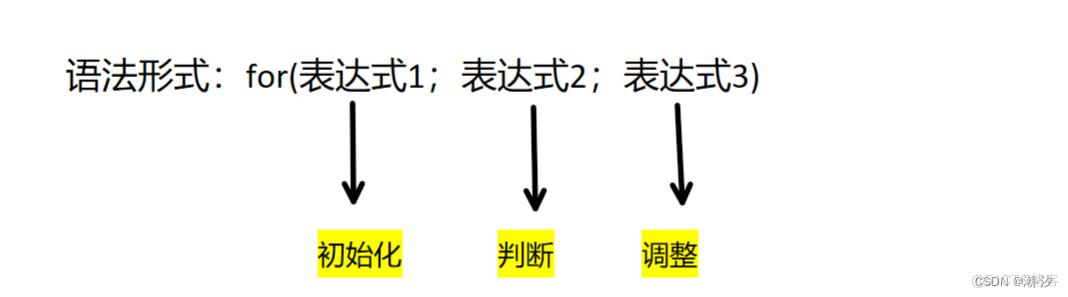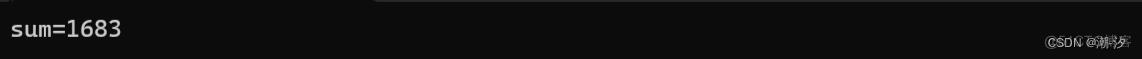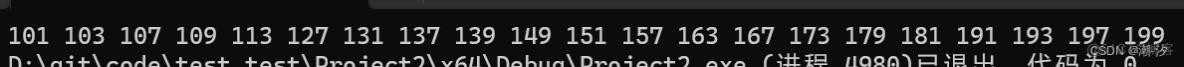﻿ 分支和循环（详解—if语句，switch语句，while循环，for循环，还有break和continue语句，goto语句） - 自由资讯

# 分支和循环（详解—if语句，switch语句，while循环，for循环，还有break和continue语句，goto语句）

1.分支 1.1 if语句 0为假（表达式为假），语句不执行非零为真（表达式成立），语句执行 例如： 503 返回1； 1320 返回0 1.1.1 else问题 if…else语句中，else后面不能直接跟括号 int main(){int

## 1.分支

### 1.1 if语句

0为假（表达式为假），语句不执行 非零为真（表达式成立），语句执行

#### 1.1.1 else问题

• if…else语句中，else后面不能直接跟括号
``````int main()
{
int a = 0;
scanf("%d", &a);
if (a > 0)
{
if (a % 2 == 0)
printf("%d是偶数", a);
else
printf("%d是奇数", a);
}
else if (a <= 0)
printf("%d不是整数", a);
return 0;
}``````

``````if (a % 2 == 0)
printf("%d是偶数", a);
else
printf("%d是奇数", a);``````

• 悬空else问题

`else`总是跟最接近的`if`匹配

！（逻辑反运算符）

&&（与运算符）

### 1.2 switch（表达式）

``````int main()
{
int a = 0;
scanf("%d", &a);
switch (a%3)
{
case 0:
printf("整数");
break;
case 1:
printf("余数是1");
break;
case 2:
printf("余数是2");
break;
}
return 0;
}``````

#### 1.2.1 switch中的default

``````int main()
{
int a = 0;
scanf("%d", &a);
switch (a)
{
case 1:
case 2:
case 3:
case 4:
case 5:
printf("工作日");
case 6:
case 7:
printf("周末");
default:
printf("输入错误");

}
return 0;
}``````

default的作用就是switch语句里所有的case都不成立时所要执行的语句。 default是用在switch语句中，提示输入错误

## 2.循环

### 2.1 while循环

``````int main()
{
int a = 0;
scanf("%d", &a);
while (a !=0)
{
printf("%d ", a % 10);
a=a / 10;
}
return 0;
}``````

### 2.2 for循环`for`循环打印1~10

``````int main()
{
int a = 0;
for (a = 1; a <= 10; a++)
{
printf("%d ", a);
}
return 0;
}``````

``````int main()
{
int a = 0;
int sum = 0;
for (a = 1; a <= 100; a++)
{
if (a % 3 == 0)
sum += a;
}
printf("sum=%d\n", sum);
return 0;
}``````## 3.break和continue语句

break是终止循环，跳出一层循环（只能跳一层） continue是跳过本次循环的代码

### 4.练习

``````int main()
{
int a = 0;
for (a = 100; a <= 200; a++)
{
int tmp = 1;
int i=0;
for (i = 2; i < a; i++)
{
if (a % i == 0)
{
tmp = 0;
break;
}

}
if (tmp == 1)
printf("%d ", a);
}
return 0;
}``````### 5.goto语句

``goto error``
【感谢龙石数据资产管理和维护 http://www.longshidata.com/pages/government.html】

﻿